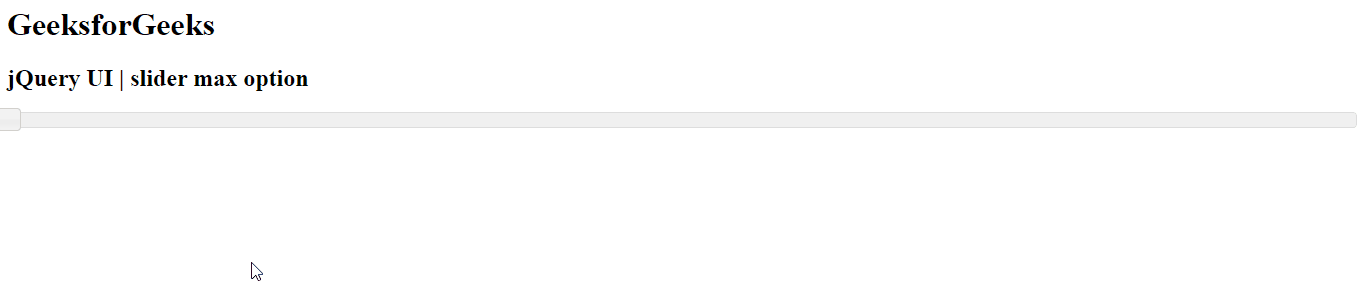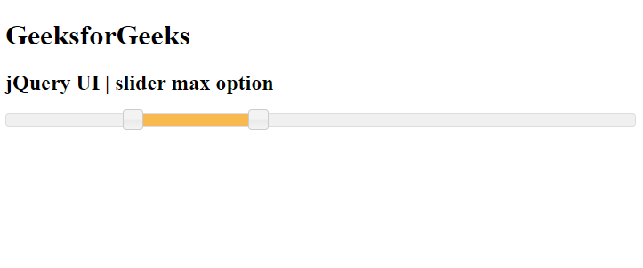# jQuery UI Slider max Option

• Last Updated : 02 Mar, 2021

jQuery UI consists of GUI widgets, visual effects, and themes implemented using jQuery, CSS, and HTML. jQuery UI is great for building UI interfaces for the webpages. jQuery UI provides us a slider control through the slider widget. Slider helps us to get a certain value using a given range. In this article, we will see how to set the max option in a slider. The max option is used to set the upper range of the slider.

Syntax:

```\$(".selector").slider(
{ max : 20}
);```

Parameters: This option accepts one parameter as discussed below.

• number: The upper range of the slider to be set.

<link href = “https://code.jquery.com/ui/1.10.4/themes/ui-lightness/jquery-ui.css” rel = “stylesheet”>
<script src = “https://code.jquery.com/jquery-1.10.2.js”></script>
<script src = “https://code.jquery.com/ui/1.10.4/jquery-ui.js”></script>

Example 1: In this example, we will be using a max number value as 15.

## HTML

 ```<``html` `lang` `= ``"en"``>``   ``<``head``>``      ``<``meta` `charset` `= ``"utf-8"``>``      ``<``link` `href` `= ``"https://code.jquery.com/ui/1.10.4/themes/ui-lightness/jquery-ui.css"``         ``rel` `= ``"stylesheet"``>``      ``<``script` `         ``src` `= ``"https://code.jquery.com/jquery-1.10.2.js"``>``      ````      ``<``script` `         ``src` `= ``"https://code.jquery.com/ui/1.10.4/jquery-ui.js"``>``      ```` ` `      ``<``script``>``         ``\$(function() {``            ``\$( "#gfg" ).slider(``               ``{max : 15}``            ``);``         ``});``      ````   ````    ` `   ``<``body``>``      ``<``h1``>GeeksforGeeks ``      ``<``h2``>jQuery UI | slider max option``      ``<``div` `id``=``"gfg"``>``   `````

Output:Example 2: In this example, we will be using the range option with min, max number value as 0 and 500.

## HTML

 ```<``html` `lang` `= ``"en"``>``   ``<``head``>``      ``<``meta` `charset` `= ``"utf-8"``>``      ``<``link` `href` `= ``"https://code.jquery.com/ui/1.10.4/themes/ui-lightness/jquery-ui.css"``         ``rel` `= ``"stylesheet"``>``      ``<``script` `src` `= ``"https://code.jquery.com/jquery-1.10.2.js"``>``      ````      ``<``script` `src` `= ``"https://code.jquery.com/ui/1.10.4/jquery-ui.js"``>``      ````      ``<``script``>``         ``\$(function() {``          ``\$( "#gfg" ).slider({``              ``range: true,``              ``min: 0,``              ``max: 500,``              ``values: [ 100, 300 ],``           ``});``         ``});         ``      ````   ````    ` `   ``<``body``>``      ``<``h1``>GeeksforGeeks ``      ``<``h2``>jQuery UI | slider max option``      ``<``div` `id` `= ``"gfg"``>``   `````

Output:My Personal Notes arrow_drop_up